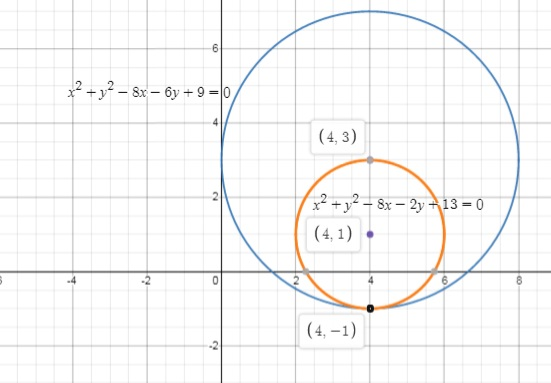# Find the equation of a circle whose center is atSarah-Louise Prince 2022-03-03 Answered
Find the equation of a circle whose center is at (4, 1) and tangent to the circle ${x}^{2}+{y}^{2}-8x-6y+9=0$. Draw the circles.
You can still ask an expert for help

• Questions are typically answered in as fast as 30 minutes

Solve your problem for the price of one coffee

• Math expert for every subject
• Pay only if we can solve itzakrwinpfo

We have to find the equation of circle having a center (4,1) and tangent to the circle ${x}^{2}+{y}^{2}-8x-6y+9=0$
The equation of the given circle is ${x}^{2}+{y}^{2}-8x-6y+9=0$
$⇒{x}^{2}-8x+16-16+{y}^{2}-6y+9-\text{⧸}9+\text{⧸}9=0$
$⇒{\left(x-4\right)}^{2}+{\left(y-3\right)}^{2}-16=0$
$⇒{\left(x-4\right)}^{2}+{\left(y-3\right)}^{2}=16⇒{\left(x-4\right)}^{2}+{\left(y-3\right)}^{2}={4}^{2}$
center (4,3) and radius $R=4$
Now we have to circles having centers
both circles touch each other,
then ${C}_{1}{C}_{2}=\sqrt{{\left(4-4\right)}^{2}+{\left(3-1\right)}^{2}}$
${C}_{1}{C}_{2}=\sqrt{\left(0{\right)}^{2}+\left(2{\right)}^{2}}$
${C}_{1}{C}_{2}=\sqrt{4}$
${C}_{1}{C}_{2}=2$
Since ${C}_{1}{C}_{2}
so the point (4,1) inside the circle.
let radius of circle having center ${C}_{1}$ is r.
then $R-r={C}_{1}{C}_{2}$
$4-r=2$
$-r=2-4$
$-r=-2$
$r=2$
equation of circle having a center (4,1) and radius $r=2$ is
${\left(x-4\right)}^{2}+{\left(y-1\right)}^{2}={2}^{2}$
${x}^{2}-8x+16+{y}^{2}-2y+1=4$
${x}^{2}+{y}^{2}-8x-2y+17=4$
${x}^{2}+{y}^{2}-8x-2y=4-17$
${x}^{2}+{y}^{2}-8x-2y+13=0$ (required equation of circle)